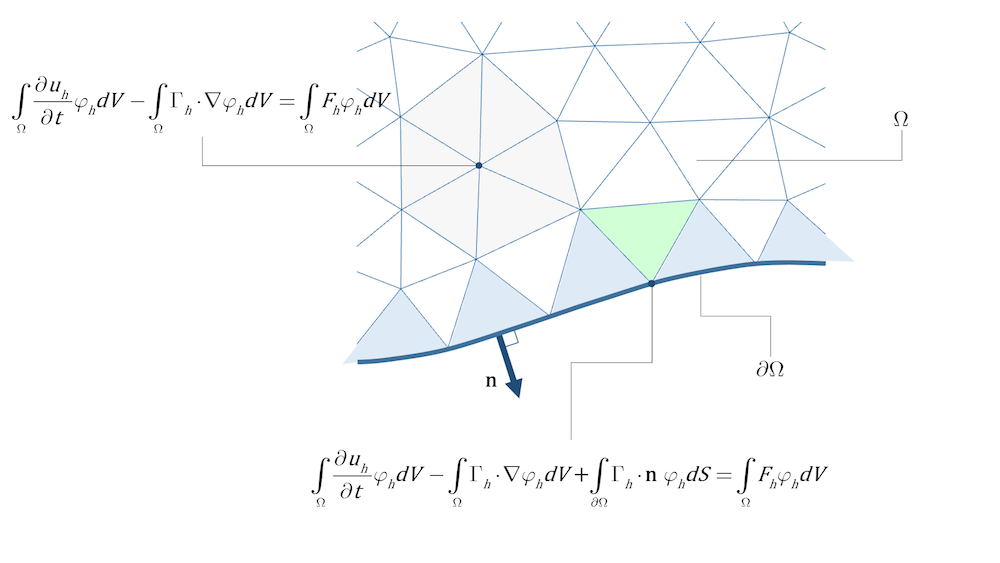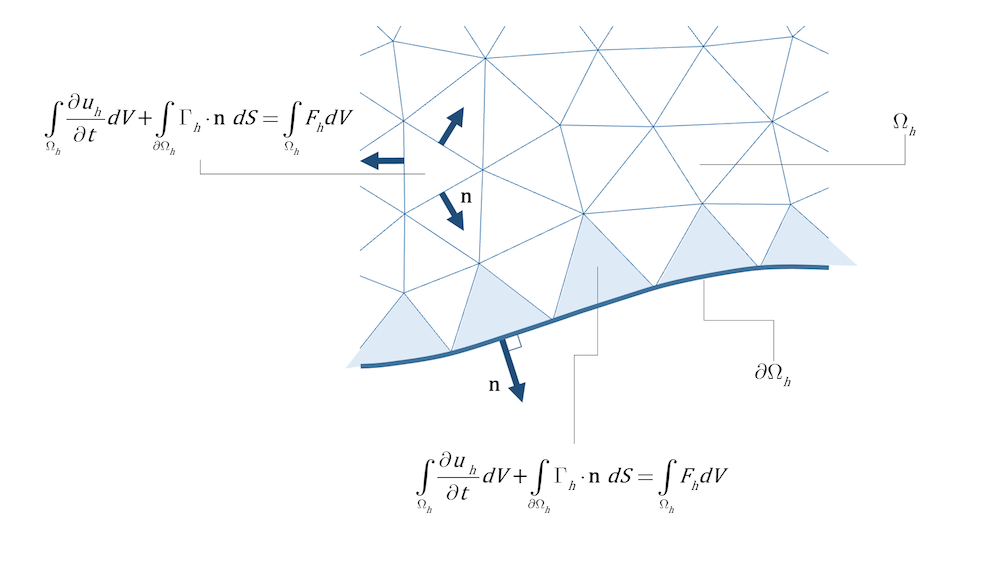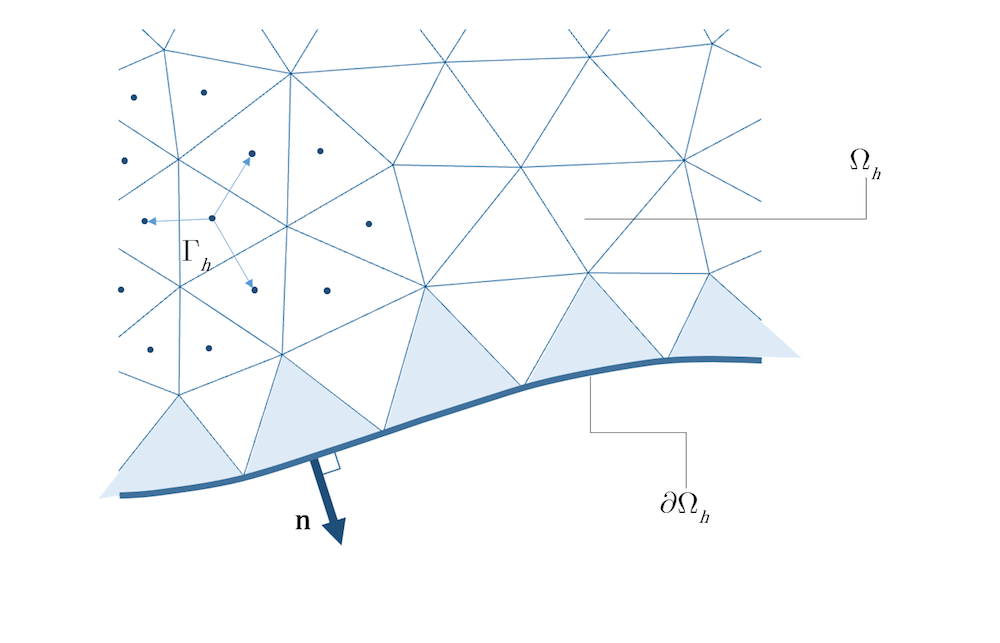# 有限元法（FEM）vs. 有限体积法（FVM）

2018年 11月 29日

### 有限元法 vs. 有限体积法：哪个最好？

#### 数学模型和数值模型

(1)

P(\frac{\partial}{\partial x},\frac{\partial}{\partial t}) u = F

(2)

$\begin{gathered} u = f{\text{ initial condition}} \hfill \end{gathered}$

$\begin{gathered} Bu = g{\text{ boundary condition}} \hfill \end{gathered}$

(3)

${P_h}{u_h} = {F_h}$

(4)

$\begin{gathered} {u_h} = {f_h}{\text{ initial condition}} \hfill \end{gathered}$
$\begin{gathered} {B_h}{u_h}= {g_h}{\text{ boundary condition}} \hfill \end{gathered}$

(5)

$\tau = \left( {P-{P_h}} \right)u$

(6)

e=\left \| {u-{u_h}} \right \|

(7)

\left \| {u-{u_h}} \right \| \to 0{\text{ as }}h \to 0$离散化的精确度 p 揭示数值解随着 h 减小收敛为精确解的速度。 (8)$e\left( h \right) \leqslant {C_1}
{h^p}
$p 值越大，近似值收敛得越快。 那么，有限元法与有限体积法在精确度上有什么内在的区别吗？通过增加基函数的阶数，理论上我们可以用有限元法达到任意精确度（在实践中，还有其他限制因素）。最常见的有限元法是二阶至三阶精度，而有限体积法是一阶至二阶精度。 #### 两者有哪些相同点和不同点呢？ 我们来看一个通量平衡方程，它是流体流动数学模型的基础： (9) \frac{\partial u} {\partial t} + \nabla \cdot \Gamma = F{\text{ in }}\Omega 在这个方程中，u 表示守恒物理量，如动量或质量，\Gamma 表示该量的通量，比如单位面积和单位时间内流过控制面的动量。 有限元法首先建立一个积分方程，方程用试函数 \varphi 加权，然后通过对模型域进行积分来求平均值： (10)$\int\limits_\Omega \frac{\partial u}{\partial t}\varphi dV + \int\limits_\Omega {\left(
{\nabla \cdot \Gamma } \right)\varphi dV} = \int\limits_\Omega {F\varphi dV} $不过，在继续之前，我们要对{\Gamma \varphi } 应用散度定理，从而得出如下表达式： (11) \int\limits_\Omega {\nabla \cdot \left( {\Gamma \varphi } \right)dV = } \int\limits_{\partial \Omega } {\left( {\Gamma \varphi } \right) \cdot {\mathbf{n}}dS} 其中，\partial \Omega 表示域 \Omega 的边界， \mathbf{n} 表示域边界的法矢。对上述方程左边部分求积分得出： (12) \int\limits_\Omega {\nabla \cdot \left( {\Gamma \varphi } \right)dV = } \int\limits_\Omega {\left( {\nabla \cdot \Gamma } \right)\varphi dV + } \int\limits_\Omega {\Gamma \cdot \nabla \varphi dV} 这意味着，根据 方程 11，我们可以得到： (13) \int\limits_\Omega {\left( {\nabla \cdot \Gamma } \right)\varphi dV + } \int\limits_\Omega {\Gamma \cdot \nabla \varphi dV} = \int\limits_{\partial \Omega } {\left( {\Gamma \varphi } \right) \cdot {\mathbf{n}}dS} 从而得出如下表达式： (14) \int\limits_\Omega {\left( {\nabla \cdot \Gamma } \right)\varphi dV} = -\int\limits_\Omega {\Gamma \cdot \nabla \varphi dV} + \int\limits_{\partial \Omega } {\left( {\Gamma \varphi } \right) \cdot {\mathbf{n}}dS} 我们可以将上面的方程 14 代入方程 10 的第二项，这样做是为了在积分方程中自然地包含通量边界条件，这种做法在随后的数值实现过程中带来好处，即通量矢量不必是可微分的，进而得出有限元法中的基础方程： (15)$\int\limits_\Omega {\frac{\partial u}{\partial t}\varphi dV}-\int\limits_\Omega {\Gamma \cdot \nabla \varphi dV} {\text{ + }}\int\limits_{\partial \Omega } {\Gamma \cdot {\mathbf{n}}{\text{ }}\varphi dS} = \int\limits_\Omega {F\varphi dV} $这就是所谓的弱形式。左边的第三项对u\Gamma 在域\partial \Omega 边界上的通量进行积分。如果弱形式对大量的试函数\varphi 都成立，则它只与物理模型有关。常见的一种选择是使用多项式，但也可以是其他类型的函数。一个特例是常数试函数；例如，\varphi = 1。上面最后一个方程方程 15 变为： (16)$\int\limits_\Omega {\frac{\partial u}{\partial t}dV} {\text{ + }}\int\limits_{\partial \Omega } {\Gamma \cdot {\mathbf{n{\text{ }}dS} = \int\limits_\Omega {F{\text{ }}dV} \$### 有限元法的前景

COMSOL 软件主要采用有限元法解决 CFD 问题，这是我们的专长所在。在过去的 15 年中，研发团队在研究采用不连续试函数和基函数的有限元法方面取得了重大成就，也就是引言中提到的 DG 方法，这些方法中的试函数对于每个element来说都是局部的，弱形式的方程适用于每个element。局部守恒是自动实现的，高阶离散化非常简单，也不需要重建解，但零阶方法除外，零阶方法相当于有限体积法。此外，element边界上的局部通量是公式的自然组成部分，这意味着很容易实现稳定性。DG 方法的一个缺点是引入了相对大量的额外自由度，这就需要研究混合 DG 方法，在这种方法中，离散化更为精简，自由度更少。

### 推荐阅读

• 有限元法（FEM）
• 用代数多重网格（AMG）法执行大型 CFD 仿真
• 借助虚构解方法验证仿真

登录 | 注册
正在加载...
浏览 COMSOL 博客